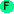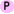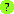Predicates

Predicates

Arc includes multiple predicates. The dead and ssyntax predicates are listed elsewhere.< arg arg [...] Less than comparison. Applies to numbers, strings, symbols, or chars. If multiple arguments are given, the sequence must be monotonically increasing. ```>(< 1 2) t ``` ```>(< 1 2 3) t ``` ```>(< 1 3 2) nil ``` ```>(< "a" "b") t ``` ```>(< 'a 'b) t ``` ```>(< #\a #\b) t ```> arg arg [...] Greater than comparison. Applies to numbers, strings, symbols, or chars. If multiple arguments are given, the sequence must be monotonically decreasing. ```>(> 1 2) nil ``` ```>(> 3 1 2) nil ``` ```>(> "a" "b") nil ``` ```>(> 'a 'b) nil ``` ```>(> #\a #\b) nil ```bound symbol Tests is a symbol is bound. ```>(bound 'foo) nil ``` ```>(do (assign y 1) (bound 'y)) t ```exact value Tests if the value is an exact integer. ```>(exact 3) t ``` ```>(exact 3.14) nil ```is val [val ...] Tests equality with eqv? ```>(is 1 2) nil ``` ```>(is "a" "a") t ``` ```>(is '(1) '(1)) nil ``` ```>(is 1 1 1 1) t ``` ```>(is nil '()) t ```<= [arg ...] The less-than-or-equal predicate. It can take an arbitrary number of arguments. There is no short-circuiting; all arguments are evaluated. Arguments must be comparable. Arguments can be numbers, characters or strings, but all arguments must be of the same type. ```>(<= 2 2.0) t ``` ```>(<= #\a #\c #\e) t ``` ```>(<= "foo" "bar") nil ```>= [arg ...] The greater-than-or-equal predicate. It can take an arbitrary number of arguments. There is no short-circuiting; all arguments are evaluated. Arguments must be comparable. Arguments can be numbers, characters or strings, but all arguments must be of the same type. ```>(>= 2 3) nil ``` ```>(>= #\a #\c #\e) nil ``` ```>(>= "baz" "bar") t ```dotted x Returns true if x is a dotted list.isa x y Tests if x has type y.orf function ... Creates a function on one variable that tests if any of the original functions are true when applied to the variable.andf function ... Creates a function on one variable that tests if all of the original functions are true when applied to the variable.atend i s Tests if index i is at the end or beyond in sequence or string s.testify test Creates a predicate from test. If test is a function, it is used as the predicate. Otherwise, a function is created to test equality with test using 'is'.acons x Tests if x is of type 'cons, i.e. if x is a non-nil list.atom x Tests if x is an atom, that is anything other than type 'cons. Note that atom and acons are opposites.alist x Tests if x is a list, i.e. nil or of type 'cons. The alist and acons predicates are the same except for nil, which is a list but an atom, not acons.some test seq Tests if any element of seq satisfies test. The sequence is either a list or a string. The test is a predicate or value, which is wrapped in testify.all test seq Tests if all elements of seq satisfies test. The sequence is either a list or a string. The test is a predicate or value, which is wrapped in testify.isnt x y Tests inequality; opposite of is.iso x y Compares x and y. If they are lists, they are compared element-by-element.empty seq Tests if seq is empty. Works on lists, strings, and tables.